# Math Worksheets Land

Math Worksheets For All Ages

# Math Worksheets Land

Math Worksheets For All Ages

# Adding Denominators of 10 and 100 Worksheets

We have had some experience, at this point, with adding and subtracting fractions. We know that the general rule for adding fractions is to add the numerators, but you can only do that if the denominators are the same. This is where we begin to explore finding the sum of unlike fractions. A nice place to start is working on base ten pair value in the denominator. Hundredths and tenths are a nice play to start. In science class students should be working on measurement units that are expressed at these levels, so it really offers a great starting point for students. These worksheets and lessons help students learn how to work with operations between fractions where the denominators have values that are either tenths or hundredths.

### Aligned Standard: Grade 4 Fractions - 4.NF.5

• Answer Keys - These are for all the unlocked materials above.

### Homework Sheets

We start by adding two fractions with very different denominators. We then work with cross multiplication to find unknowns.

• Sums Homework 1 - A common denominator must be used in order to add fractions. When fractions are added, they must refer to the same whole.
• Unknowns Homework 2 - What should x equal?
• Sums Homework 3 - A common denominator must be used in order to add fractions. When fractions are added, they must refer to the same whole.

### Practice Worksheets

We follow suit here, too. We have two unknowns and one sum.

• Unknowns Practice 1 - Find the final value of the following problem.
• Unknowns Practice 3 - Find that missing value. You will still need to make sure the bottoms are the same, at first.

### Math Skill Quizzes

We mixed in both skills here. You will find them very helpful in this format.

• Quiz 1 - Some have missing values and others just want to be evaluated.
• Quiz 2 - You will process both a series of sums and balance a few equations along your travels.
• Quiz 3 - Make everything equal first before you attack it.

### How to Convert a Fraction Between a Tenth and a Hundredth

Tenths and hundredths are place values that represent a very small quantity. While they may be almost deemed insignificant by most in measures of time, they mean the difference between first at last place in many Olympic and well attended sporting events. The numbers after the decimal point come under these place values. The first digit after a decimal point is the tenths position and the number following the tenth value presumes the place value of hundredths.

Our numeracy system is entirely built around the concept of base ten meaning that as you move from one place value to the next you are either moving to a value that is ten times greater or less, based on which direction you are moving. Moving to the left is ten times greater and moving to the right is ten times less.

When we say one tenth, we are terming one part of number 10 which is 1/10 in fraction and 0.1 in decimal form. One hundredth is one part of the number 100 which is 1/100 in fraction and 0.001 in decimal form. In some mathematical situation you might be asked to convert a fraction from a value in the tenths into hundredths.

To convert a fraction from tenths into hundredths, you have to multiply the fraction by 1/(10 ). If you want to covert a whole number into tenths you multiply it by 1/10 and to convert it into hundredths, you multiply the number by 1/100.

You may also be asked to write these values in decimal form. In order to do this just remember that tenths is the first place value from the right of the decimal point and hundredths is the second place value to the right of the decimal point. Use the chart below to visualize the tens, ones, tenths, and hundredths place value and their relative position to the decimal point. Let’s visualize 74.39.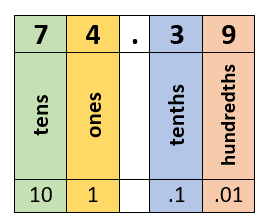### How Do You Add Fractions That Have Denominators of Tenths and Hundredths?

This is a common exercise that you will see in science laboratories. As with adding any type of fraction: convert them to the same denominator before you process the addition operation. We will work through the problem of adding 4 the following fractions to explain this concept: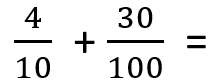These fractions do not have a common denominator. When you are adding fractions, they need to refer to the same whole value. The easiest way to make sure they have the same denominator is to convert the value in tenths to hundredths.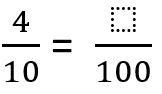When we divide 100 by 10, we get 10. This means that we must do the same thing with the 4 and multiply it by 10. This now leaves us with finding the sum of the following fractions: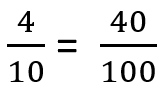We can now attempt the problem with both values having the same denominator: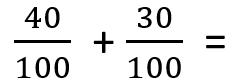In fraction addition, the denominators stay the same and we add the numerators (40 + 30 = 70). So the final answer would be: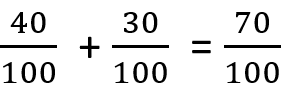Unlock all the answers, worksheets, homework, tests and more!
Save Tons of Time! Make My Life Easier Now

## Thanks and Don't Forget To Tell Your Friends!

I would appreciate everyone letting me know if you find any errors. I'm getting a little older these days and my eyes are going. Please contact me, to let me know. I'll fix it ASAP.